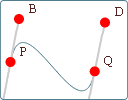Search IntMath
Close

# IntMath Newsletter: Bezier curves interactive, KinectMath, puzzles

By Murray Bourne, 17 Jan 2013

17 Jan 2013

1. Interactive Bezier Curve Graphs
2. Kinect Math
3. IntMath polls
4. HOTmaths revisited
5. Math puzzles
6. Friday math movie
7. Final thought - Questions for learning

Happy New Year everyone!

In case you missed it, 2013 is year of Mathematics of Planet Earth. Events will include public lectures like "The challenge of sustainability and the promise of mathematics" by Princeton University professor Simon Levin in Melbourne, Australia on 29th Jan 2013.

In math class we should be making better use of math students' time and energy to plan for a more sustainable, and happier future.

## 1. Interactive Bezier Curve GraphsHere's an interactive graph where you can explore straight lines, parabolas, cubics and Bezier curves. You'll find it towards the bottom of this Math of Vector Art page. Interactive Bezier Curve Graphs

## 2. Kinect MathMany students prefer learning via hands-on activities. Kinect Math is an interesting approach using Microsoft's Kinect. Students interact with various graphs and other math concepts using their hands. See some videos about it here: KinectMath - A Kinesthetic Learning Experience (no longer available)

## 3. IntMath Poll: Math homework per week

The latest poll asked readers about the amount of math homework they do per week during semester. Here are the results:

More than 6 hours
36%

Between 2 and 4 hours
16%

Between 1 and 2 hours
14%

Between 4 and 6 hours
12%

Less than 1 hour
12%

None
9%

The surprise here is that over 1/5 of respondents do one hour or less For most math courses, this would be inadequate.

New poll: The latest poll asks readers whether it's important to know the history of each math topic. You can respond on any page in IntMath.

## 4. HOTmaths revisitedHOTmaths is an interesting online math resource. I take another look after a few years. Do you use it? How do you find it? HOTmaths revisited

## 5. Math puzzles

The puzzle in the last IntMath Newsletter involved finding the number of possible positive integer solutions for a given equation.

Correct answers with reasoning were given by Thomas A Buckley and Tom Barrett.

Tomas Garza fired up Mathematica, produced a 100 x 200 x 200 item table, and then used brute-force counting (which took over a minute) to find the correct rows, and from there deduced the correct answer.

A systematic approach is to consider the smallest value of y + z which occurs when y = z = 1, and if so, 2x is then n − 2. Then we can let x = 1, 2, 3 ... (n − 2)/2. Then y + z = n − 2, n − 4, n − 6, ... to which there are n − 3, n − 5, n − 7, ... solutions in positive integers. We then use the sum of an arithmetic progression formula to obtain the solution (n − 2)2/2.

New puzzle: A certain bathtub with the plug in and the tap on will fill at a uniform rate in 12 minutes. The bath will empty with the plug out at a uniform rate in 15 minutes. How long will it take to fill the bathtub if the plug is out, the tap is on, and it was originally empty?

## 6. Friday math movie: Teach statistics before calculus!This short talk argues that people should know about probability and statistics before calculus. Do you agree with him? Friday math movie: Teach statistics before calculus!

## 7. Final thought: Questions for learning

Many students do not maximize their learning outcomes. If we turn up for class with no idea what's going to happen, we just make it harder for ourselves.

Here are some things to think about before your next math class.

How have I prepared for class today? (Revised last week's key points? Understood what the formulas mean? Done the homework? Looked over what we are going to do today?)

What's the best way for me to prepare for a class like this one? (Sharing ideas with others can be a good way to pick up tips.)

What questions do I have? (If you have a good clear list of things you want to find out or understand better, it helps focus your learning. Sharing questions and answers with classmates can help to understand what is going on.)

Why did I get these exam questions wrong? (Many students just worry about the exam grade, but don't put effort into understanding what went wrong. Talking through the common errors is a good way not to repeat them.)

What do I need to do to avoid missing questions like these on the next exam? (Thinking about this will also help you focus in class. If your tests are mutliple choice, try hiding the choices before doing the problem. Too many students try to work backwards from the most likely correct answer!)

Until next time, enjoy whatever you learn.

### Comment Preview

HTML: You can use simple tags like <b>, <a href="...">, etc.

To enter math, you can can either:

1. Use simple calculator-like input in the following format (surround your math in backticks, or qq on tablet or phone):
a^2 = sqrt(b^2 + c^2)
(See more on ASCIIMath syntax); or
2. Use simple LaTeX in the following format. Surround your math with $$ and $$.
$$\int g dx = \sqrt{\frac{a}{b}}$$
(This is standard simple LaTeX.)

NOTE: You can mix both types of math entry in your comment.